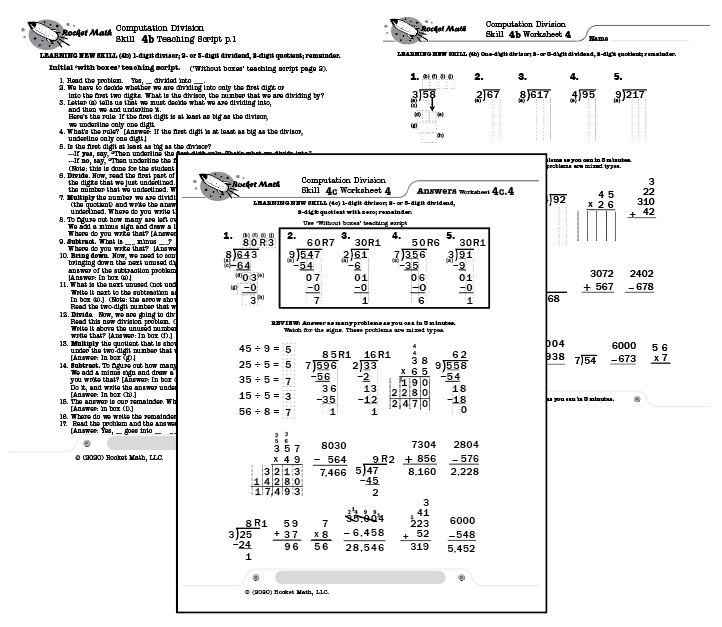## How to do (compute) multi-step division problems

After becoming fluent with division facts, the best way for students to retain the knowledge of those facts is by doing division computation.  If students have not been taught division computation, this program breaks it down into small, easy-to-learn steps that are numbered in a teaching sequence that leaves nothing to chance.

## Assessment to find which skill to teach first

There is an assessment available so you can test and see where to begin instruction. Find where the student first starts having troubles and begin teaching there.

## Skills in this Learning Track

Note that the number for each skill gives the grade level as well as indicating the teaching sequence.  Skill 3b is a 3rd grade skill and after skill 3c is learned the next in the sequence is skill 4a.  The sequence of skills is drawn from M. Stein, D. Kinder, J. Silbert, and D. W. Carnine, (2006) Designing Effective Mathematics Instruction: A Direct Instruction Approach (4th Edition) Pearson Education: Columbus, OH.

(3b) Dividing 1-digit divisor and quotient with remainder.

(3c) Division equation with ÷ sign; facts with no remainder

(4a) 1-digit divisor; 2- or 3-digit dividend, 2-digit quotient; no remainder.

(4b) 1-digit divisor; 2- or 3-digit dividend, 2-digit quotient; remainder.

(4c) 1-digit divisor; 2- or 3-digit dividend, 2-digit quotient with zero; remainder.

### not yet completed levels–to be developed upon demand–ask Dr. Don

(4d) 1-digit divisor; 3- or 4-digit dividend, 3-digit quotient.

(4e) 1-digit divisor; 3- or 4-digit dividend, 3-digit quotient with zero.

(4f) 1-digit divisor; 4- or 5-digit dividend, 4-digit quotient.

(4g) Rounding to the nearest ten.

(4h) 2-digit divisor; 1- or 2-digit quotient, all estimation yields correct quotient.

(4i) 2-digit divisor with incorrect estimated quotients.

## How to teach this Learning Track

For each skill there is a suggested Teaching Script giving the teacher/tutor/parent consistent (across all the skills we use the same explanation) language of instruction on how to do the skill.  The script helps walk the student through the computation process.  For the teacher, in addition to the script, there are answer keys for the five worksheets provided for each skill.

Each worksheet is composed of two parts.  The top has examples of the skill being learned that can be worked by following the script.  After working through those examples with the teacher the student is then asked to work some review problems that are already known.  The student is asked to do as many as possible in 3 minutes—a kind of sprint.  If all is well the student should be able to do all the problems or nearly all of them, but finishing is not required.  Three minutes of review is sufficient for one day.

There are five worksheets for each skill.  Gradually as the student learns the skill the teacher/tutor/parent can provide progressively less help and the student should be able to do the problems without any guidance by the end of the five worksheets.  There are suggestions for how to give less help in the teaching scripts.